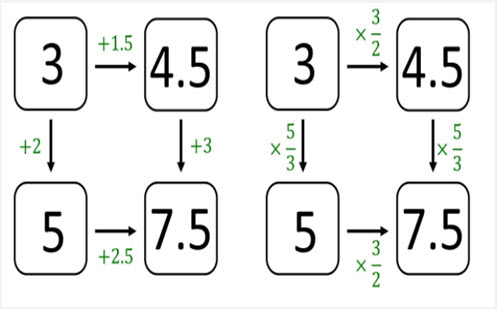Go to website

# Ratio connections

This ratio inquiry is about making connections, generating examples, and finding patterns and rules. The inquiry encourages students to regulate their own activity while exploring a prompt (an equation, statement or diagram). It involves students in questioning, analysing, conjecturing and generalising. Students can create their own examples using the template or prompt sheets provided. Teachers can make reference to the PowerPoint presentation provided on Ratio Inquiry.

Year level(s) Year 7
Audience Teacher
Teaching strategies and pedagogical approaches Feedback, Questioning, Collaborative learning, Differentiated teaching, Mathematics investigation, Metacognitive strategies, Worked examples
Keywords multipier, reciprocal, multiplicative relationships, multiplier, ratio

## Curriculum alignment

Curriculum connections English, Numeracy, Critical and creative thinking
Strand and focus Algebra, Number, Apply understanding, Build understanding
Topics Addition and subtraction, Decimals, Algebraic expressions, Data representation and interpretation, Integers, Multiples, factors and powers, Multiplication and division, Operating with number, Patterns and algebra, Proportion, rates and ratios
AC: Mathematics (V9.0) content descriptions
AC9M7N08
Recognise, represent and solve problems involving ratios

Numeracy progression Interpreting fractions (P8)
Multiplicative strategies (P9)
Proportional thinking (P3)